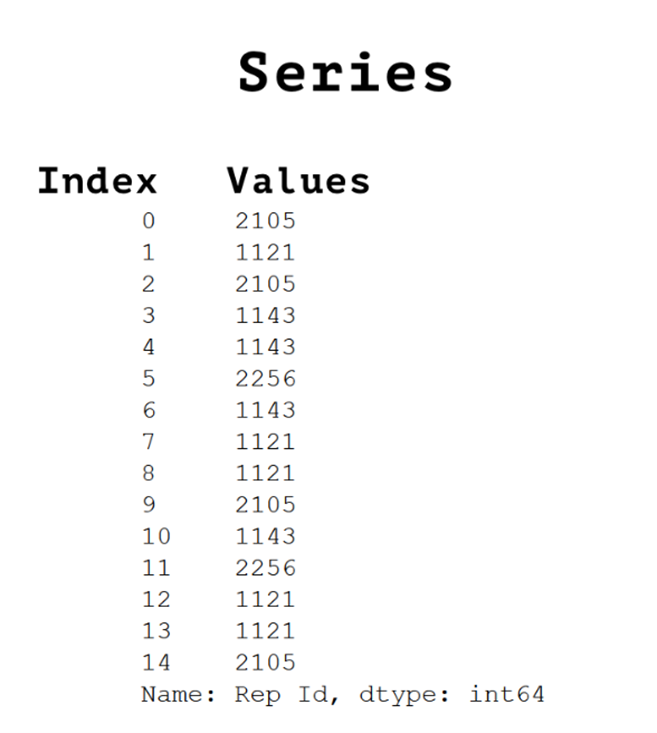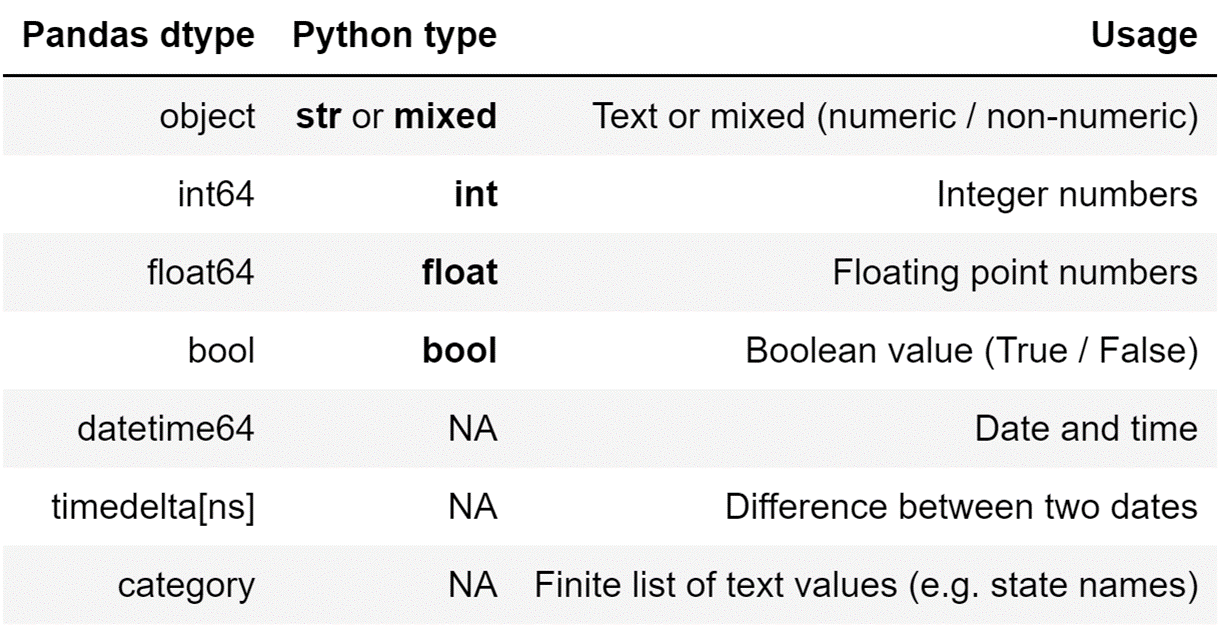# Intro to Pandas: What Are Pandas Series Objects?

When covering the Pandas package, most focus on Pandas DataFrames, but that way of approaching the Pandas package is flawed.
By Boris Delovski • Updated on Oct 23, 2022<< Read the previous article in the series: Intro to Pandas: What is Pandas in Python?

In this article, I will focus more on Pandas Series objects. When covering the Pandas package, most focus on Pandas DataFrames immediately, but that way of approaching the Pandas package is flawed. As with everything else, it is a much better idea to start from the basics to build a strong foundation.

Like how it is a much better idea to learn about NumPy before learning about Pandas, I will also focus on Pandas Series before I move on to Pandas DataFrames. I will cover what a Pandas Series is, how you can create Pandas Series objects, what the different Pandas’ data types are, and how you can access values stored inside of a Pandas Series object.

I will however leave some more advanced topics for when I talk about DataFrames, because those topics are more relevant in the context of the Pandas Series objects being columns of Pandas DataFrames.

Article continues below

## What is a Pandas Series?

A Pandas Series is the basic data structure in Pandas. It is one-dimensional and it consists of an array of data (values), and an array of labels (indices). As the most basic element in Pandas, a Pandas Series is also the building block of Pandas DataFrames. You can think of a Pandas Series as a column of a Pandas DataFrame.

A Pandas Series can contain data of multiple types, but typically when you are building a Pandas DataFrame you’ll want the data in the columns of a DataFrame to be homogenous. Which means that it is preferable to have data of one type in a Pandas Series.Image Source: Screenshot of a Pandas Series, Edlitera

## How to Create a Pandas Series

Pandas Series objects are very easy to create. You create them from Python data types that serve as collections of data (lists, dictionaries, etc.).  The easiest way to create a Pandas Series is from a list by using the Series constructor from Pandas.

Here’s a typical example of creating a Pandas Series from a list:

``data = pd.Series( ['a', 'b', 'c'] )``

The previous code will create a Pandas Series that looks like this:

``````0    a
1    b
2    c
dtype: object
``````

One thing that you may immediately notice is that, by default, you are given what Pandas decided as the data type of that Series. Essentially, since you want your data in a Pandas Series to be homogenous, Pandas will always look at the data that is stored inside a Pandas Series and decide what type of data it is.

The Pandas package does, however, define its data types:Image Source: Screenshot of Pandas defined Datatypes, Edlitera

In my example above, because I’m storing strings in my Pandas Series, the Pandas Series will automatically decide that the data type stored inside is of the object data type.

This can be easily proven by accessing the dtype attribute of a Pandas Series:

``data.dtype``

On the other hand, a Pandas Series will not be automatically assigned a name. If you want to assign a name to a Pandas Series, you need to input a value to the Series constructor for the name parameter:

``data = pd.Series( ['a', 'b', 'c'], name='Letters' )``

If I check my Pandas Series now, it will look like this:

``````0    a
1    b
2    c
Name: Letters, dtype: object``````

Another very important thing to note: unless you specify what you want your index to be, Pandas will automatically define your index as a numerical range. To define how you want to index your data, you can enter another list into your constructor. This second list, entered as the value for the index argument, will be used as the index of your Pandas Series.

An example of creating one such custom index is shown below:

``````data = pd.Series(
['Andorra', 'Belgium', 'Croatia', 'Albania'],
index=['a', 'b', 'c', 'a'])``````

The code above will create a Pandas Series that looks like this:

``````a    Andorra
b    Belgium
c    Croatia
a    Albania
dtype: object``````

There is an easier way to create a Pandas Series with a custom index, and that is to create it from a dictionary.

The dictionary keys become the index of your Pandas Series, and the dictionary values become the values of your Pandas Series, shown here in this example:

``````data = pd.Series({'a': 'Andorra', 'b': 'Belgium', 'c': 'Croatia', 'a': 'Albania'})
``````

This will create a Pandas Series identical to the one that I created a few moments ago, but I won't need to input any extra values to my Pandas Series constructor.

## How to Access Data in a Pandas Series Using Indexing

To access a specific value in a Pandas Series you can just reference its index. The procedure is the same for numerical indices and non-numerical indices.

Let's say that I have the following Pandas Series called countries:

``````0    Andorra
1    Belgium
2    Croatia
dtype: object``````

If I want to access the value 'Croatia,' I can simply reference its index:

``countries``

Even if the index is non-numerical, I access my data in the same way.

Let's say that I'm working with the following Pandas Series named countries_2:

``````a    Andorra
b    Belgium
c    Croatia
a    Albania
dtype: object``````

To access the value 'Croatia' in this Series, I need to call upon the index 'c' :

``countries_2['c']``

If I want to access multiple values, I can use slicing in a way very similar to how you would typically slice Python lists. I will just need to define the starting index and the finishing index inside the square brackets, and I will get multiple values from my Pandas Series.

Don't forget: the value that is assigned the starting index will be included in your slice, but the value that is assigned the finishing index of the slice won't be included in the slice.

Let's demonstrate this on the following example Pandas Series stored in the variable countries:

``````0    Andorra
1    Belgium
2    Croatia
3    Albania
dtype: object``````

If I want to access the values 'Belgium' and 'Croatia,' I can start my slice on the index value of 'Belgium' and end it on the index value of 'Albania,' because the value with the finishing index won't be included in the slice (the last value included is the one before that). I will get the two values I am interested in:

``countries[1:3]``

I can also use negative indexing when slicing, which means that I can get the same result I got above by using the following code:

``countries [-3:-1]``

Other slicing rules also apply.

To start slicing from the beginning I can leave the starting index empty. This means that I can, for example, get all of the values in the Series except the final value using the following code:

``countries [:-1]``

The same can be said for leaving the finishing index empty. This will automatically tell Pandas that I want to access all values from the starting index up to the end of the Pandas Series.

So, if I want to access the last two values, I can do it using the following code:

``countries [-2:]``

Finally, to access data at certain intervals, let's say every second value in my Pandas Series, I can use the following code:

``countries [::2]``

As you can see, since slicing Pandas Series is very similar to slicing lists, as long as you know the rules behind slicing Python lists you shouldn't have any problems accessing the data you are interested in.

There is, however, one thing you need to be very careful about: even if your index is non-numerical, slicing a Pandas Series as demonstrated previously will treat it as such.

This means that if I have the following Pandas Series stored in the variable data shown in this example below:

``````a    Andorra
b    Belgium
c    Croatia
a    Albania
dtype: object``````

I will still access the first two values as if the index was numerical:

``data[:2]``

This is why this simple type of accessing values is not used when you have a complex index. Instead you use the .loc and .iloc indexing accessors. I am going to cover those in the next article where I cover how Pandas DataFrames work, since this topic is even more relevant there. I will also demonstrate how to change values stored in a Pandas Series when I talk about Pandas DataFrames, by covering how to change a value in a Pandas DataFrame column, because you will rarely need to modify values in a Pandas Series unless that Series is a part of a DataFrame.

In this article, I covered all of the topics important for understanding what are Pandas Series objects and how they function. Knowing these basics is very important for understanding how multiple Pandas Series objects come together to form a Pandas DataFrame object.

What I didn't cover is exactly how multiple Pandas Series objects interact together and how you can modify them. These are topics best left for when I talk about Pandas DataFrames in the next article, because some of the concepts only become truly important once you start treating Pandas Series objects in the context of being columns of Pandas DataFrame objects. When I start explaining Pandas DataFrames, I will also cover manipulating DataFrame columns, and since a DataFrame column is nothing more than a Series object you can, if you need to, apply everything you learn about modifying columns to Pandas Series objects.# Boris Delovski

Boris is a data science trainer and consultant who is passionate about sharing his knowledge with others.

Before Edlitera, Boris applied his skills in several industries, including neuroimaging and metallurgy, using data science and deep learning to analyze images.